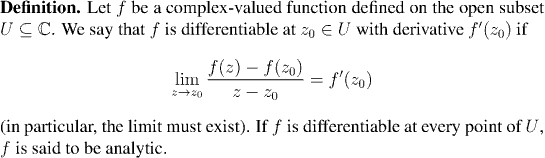Analysis WebNotes
arrow_back

## Complex differentiation

When you have a complex-valued function of a real or complex variable, we can talk about differentiating it in exactly the same way as when the function is a real-valued function of a real variable. You can form the difference quotient in exactly the same way, and if the limit of the difference quotient exsists at a particular point we call that the value of the derivative at that point.Remark:
Although we shall not study analytic functions in this course, the class of analytic functions is extremely important in analysis, and throughout mathematics. There is a very beautiful and complete theory of analytic function which is developed in the area of mathematics called complex analysis.

Just to whet your appetite, here are some of the amazing results which can be proved about analytic functions:

• If a function is analytic then its derivative is also analytic (this is is stark contrast to the case of real-valued functions)
• Every analytic function can be expressed in terms of a power series
• Analytic functions are determined by their "boundary values": if you have an analytic function on the unit disk, then its values on the unit circle determine its behavior inside the disk as well (and the values inside the disk can be expressed in terms of the values on the boundary by a beautiful formula involving integration).
When f is a function which is defined on a region of the complex plane which includes points of the real line, then one can talk about both the complex and the real derivative of f. The complex and the real derivative both use the same formula for the difference quotient. The difference between them is that for the complex derivative, z approaches zo through both real and complex values. For the real derivative, only real values of z are considered. Thus, if the complex derivative of a function exists at a point in the real line, then the real derivative also exists, and the two are equal. The converse is not true.

For our purposes, this will mean that anything we say about complex derivatives of power series when z is real will equally well hold for the real derivative.There are versions of Proposition 4.8 and Proposition 4.9 for complex differentiation that look formally exactly like their real analogues.

In fact the proofs are the same too; look back at the proofs of those results and you'll see that there wasn't anywhere we did anything that wouldn't have worked just as well in the complex plane.